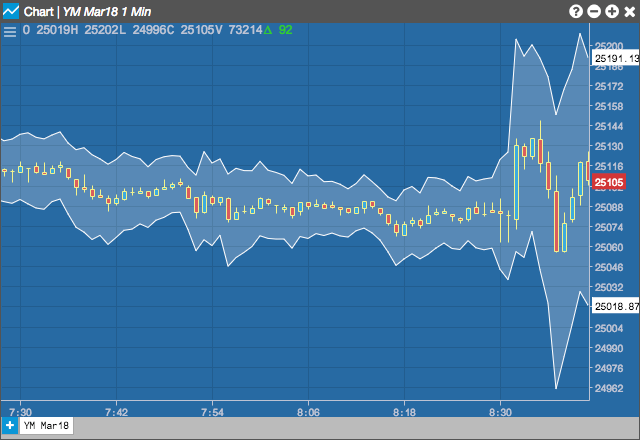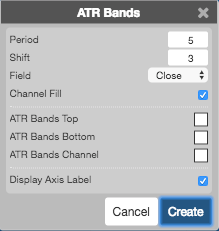Charts

# ATR Bands

The ATR Bands indicator shows trends in price movements. Based on the average true range (ATR), the ATR bands are plotted around the ATR values to indicate the direction of movements in price.## Configuration Options• Period: Number of bars to use in the calculations.
• Shift: Number of ATR multiples to draw the bands above and below the ATR.
• Field: Price or combination of prices to use as the base for average calculations. Possible values include:
• Open
• High
• Low
• Close
• HL/2 $$\left ( \frac{High + Low}{2} \right )$$
• HLC/3 $$\left ( \frac{High + Low + Close}{3} \right )$$
• HLCC/4 $$\left ( \frac{High + Low + Close + Close}{4} \right )$$
• OHLC/4 $$\left ( \frac{Open + High + Low + Close}{4} \right )$$